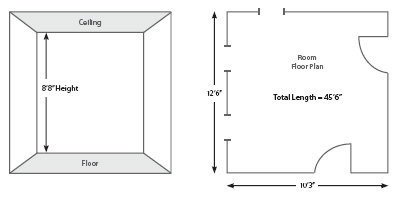space

## Installation

How much do I need?
Professional Installation

How much do I need?

Just like fabrics, each production batch or “dye-lot” of wallcovering may have minor colour variations from other dye-lots.  For a perfect job all your wallcovering should ideally be from the same dye-lot so we print the “dye-lot number” on the label of each bolt. Ensure all rolls have the same Lot #.

To determine how much wallcovering to buy:

1. Estimate the number of square feet required.

• Measure the ceiling height and “round up” to the next foot.

• Measure the total length of all the walls to be covered and “round up” to the next foot.• Multiply Height x Total Length = square feet (sf) to be covered.
e.g.
Ceiling height 8'8”, total length of all walls is 45’ 6”.
Call it 9’ x 46’ = 414 square feet.
• Deduct 24 square feet for each door or window.
e.g. 2 doors and 3 windows, deduct 120 square feet.
Remainder is 295 square feet.
• Then add 5% for waste and pattern matching.

e.g. Call it 15 square feet.
Total is 309 square feet.

2. Divide the number of square feet to be covered by the number of square feet in a  roll.

• “Round up” to the next whole number.

• This will give the number of rolls required,
e.g. 309 square feet divided by 54 square feet per bolt = 5.7.
Round up to 6 bolts.

For a rough estimate on determining how much wallpaper is required, please see the chart below. One Bolt = 2 Single Rolls.Note – to simplify calculations for door and window allowances, deduct 1/2 bolt for each normal size door or window. Make allowances for design repeat and wastage.

BORDERS

Most spooled borders come in 5 yard rolls, which is equivalent to 15 feet.  Check the product label for exact dimensions.  Divide the yardage in the spool into the room diameter to determine the number of rolls needed.